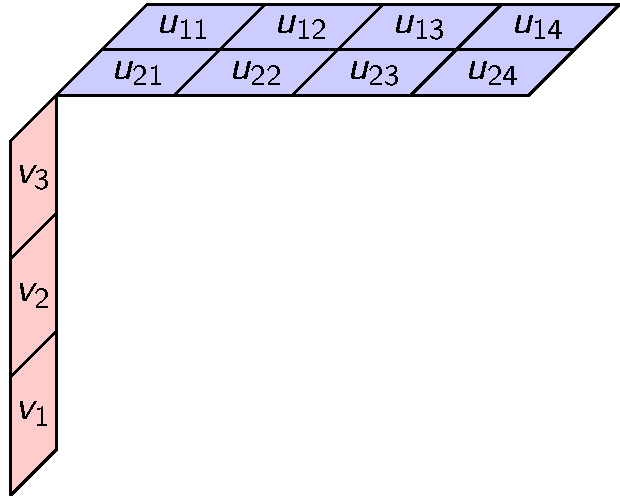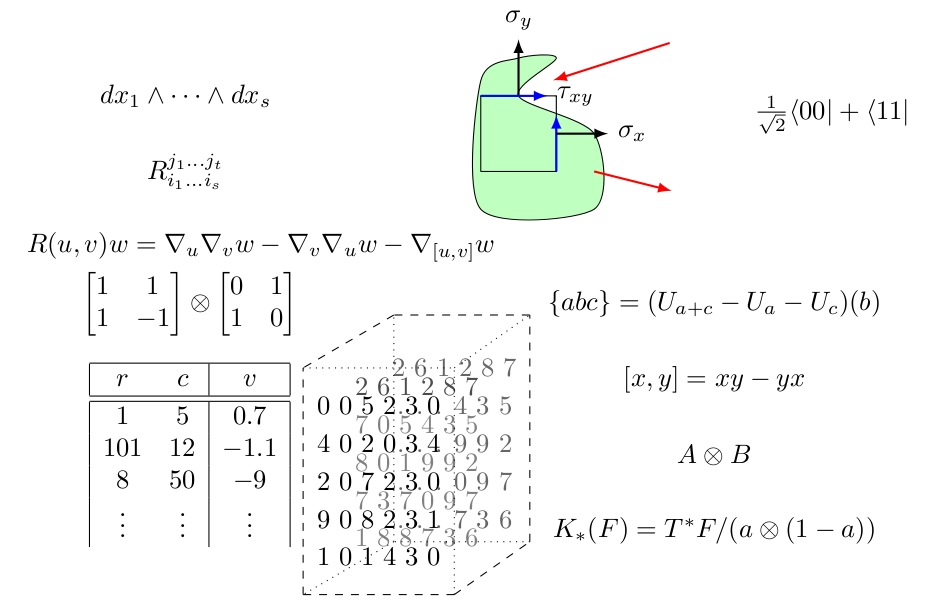# Articles### Whitney Tensor Product $\otimes$ done different### 120 years of tensors

Era 1860 1900 1940 1980 2020
Tensors are … Lengths Forms Froms & Spaces Grids, Forms, Spaces, & Multilinear maps …all things distributive.
Used by… Hamilton Geometry, Physics Geometry, Kinematics, Relativity, Quantum Physics, Algebra, Analysis Finite Geometry, Differential Geometry, Kinematics, Relativity, Quantum Mechanics, Representations, Algebras, PDEs, Categories, Model Theory, Informatics, Statistics Finite Geometry, Differential Geometry, Kinematics, Relativity, Quantum Mechanics, Representations, Algebras, PDEs, Sensors, Categories, Morley Rank, Isomorphism, Informatics, Statistics, Big Data, Machine Learning, Compression, Data Structures, Clustering, Decompositions, Dimension Reduction, Image Processing, Synthizing Data, Scanning,…and all the uses of which we haven’t a clue!### What Is a Tensor? What is a Tensor Space?### The Waring Support Rank of the Elementary Symmetric Polynomials

Definition. The Waring rank of a homogeneous polynomial $f \in C[x_1, \ldots, x_n]_d$ is the minimum $r$ such that

$f = \ell_1^d + \cdots + \ell_r^d$

for some linear forms $\ell_1, \ldots, \ell_r \in C[x_1, \ldots, x_n]_1$.

### Recovering Information in Noise

Cristopher Moore Santa Fe Institute

### Projective Varieties

Takunari Miyazaki (Trinity College, Hartford, Connecticut)

### Min-Rank

Takunari Miyazaki (Trinity College, Hartford, Connecticut,)### Shrunk subspaces of alternating matrix tuples

Let $p\geq 3$ be a prime. Let $\Lambda(n,p)$ be the set of alternating matrices over $\mathbb{F}_p$, where $A\in M(n,p)$ is alternating if for all $v\in\mathbb{F}_p^n$, $v^tAv=0$. Let $\mathbb{A}\in\Lambda(n,q)^m$ be an $m$-tuple of alternating matrices. We say two $m$-tuple of alternating matrices $\mathbb{A}_1$ and $\mathbb{A}_2$ pseudo-isometric, if there exists $T\in \text{GL}(n,p)$ such that $\langle T^t\mathbb{A}_1T\rangle=\langle\mathbb{A}_2\langle$ (as subspaces), where $\langle \mathbb{A} \rangle$ denotes the linear space spanned by alternating matrices in the $m$-tuple $\mathbb{A}$.